Homework Help Question & Answers

# Perform the indicated operation and write the answer in the form a +bi, where a and...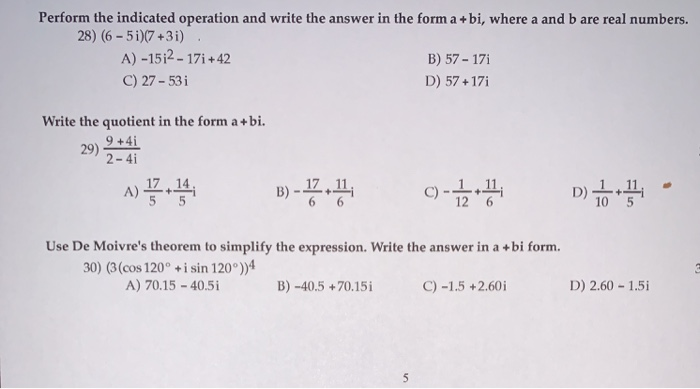Perform the indicated operation and write the answer in the form a +bi, where a and b are real numbers. 28) (6-5i)(7 +3i) A) -15;2 - 171 +42 B) 57 - 171 C) 27 - 531 D) 57 +171 Write the quotient in the form a +bi. 9 +41 29) 2-4i B) -1.4, C) -1 5 Use De Moivre's theorem to simplify the expression. Write the answer in a +bi form. 30) (3 (cos 120° + i sin 120°))4 A) 70.15 - 40.51 B) -40.5 +70.151 C) -1.5 +2.60i D) 2.60 - 1.51

#### Homework Answers

Answer #1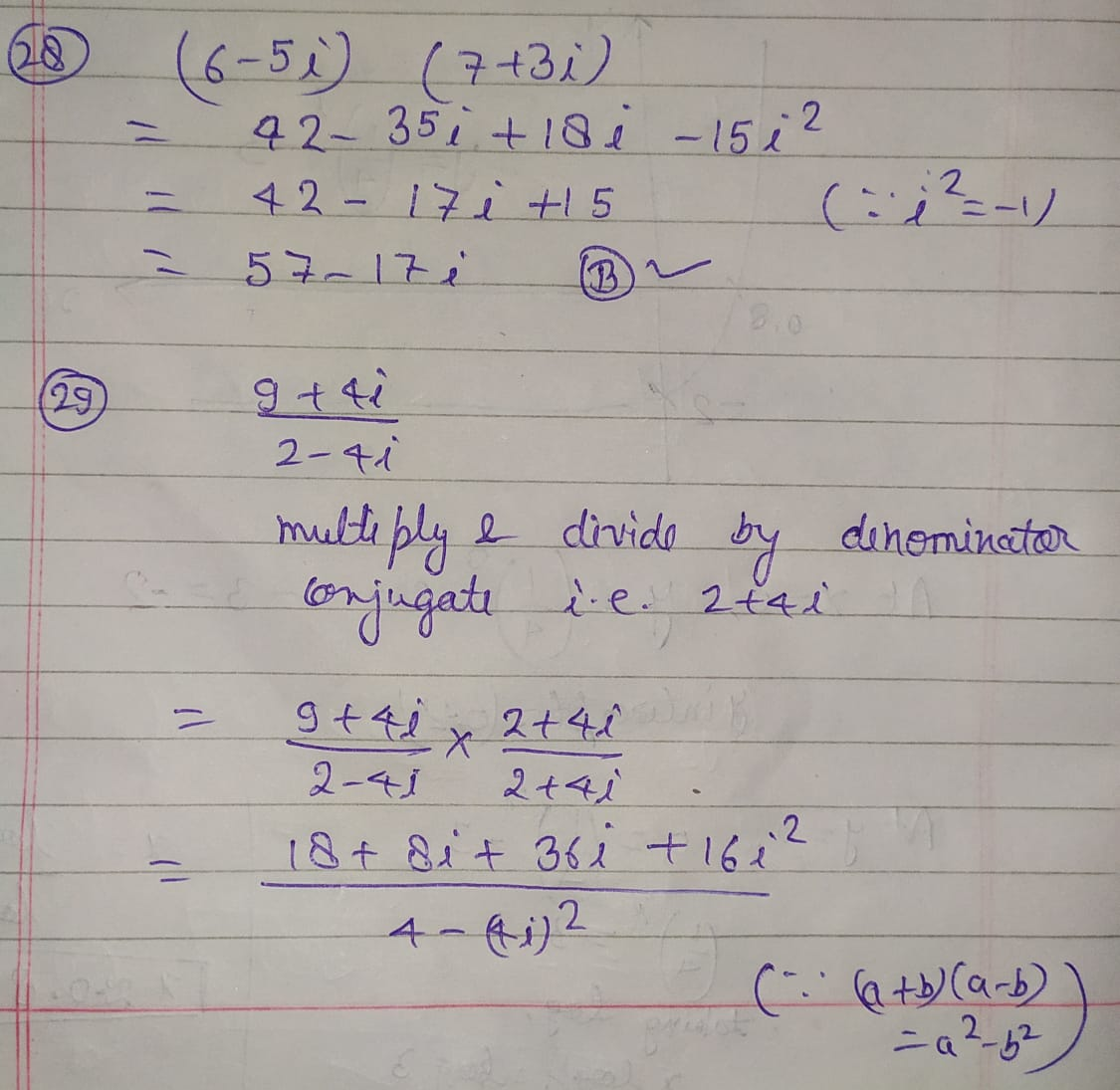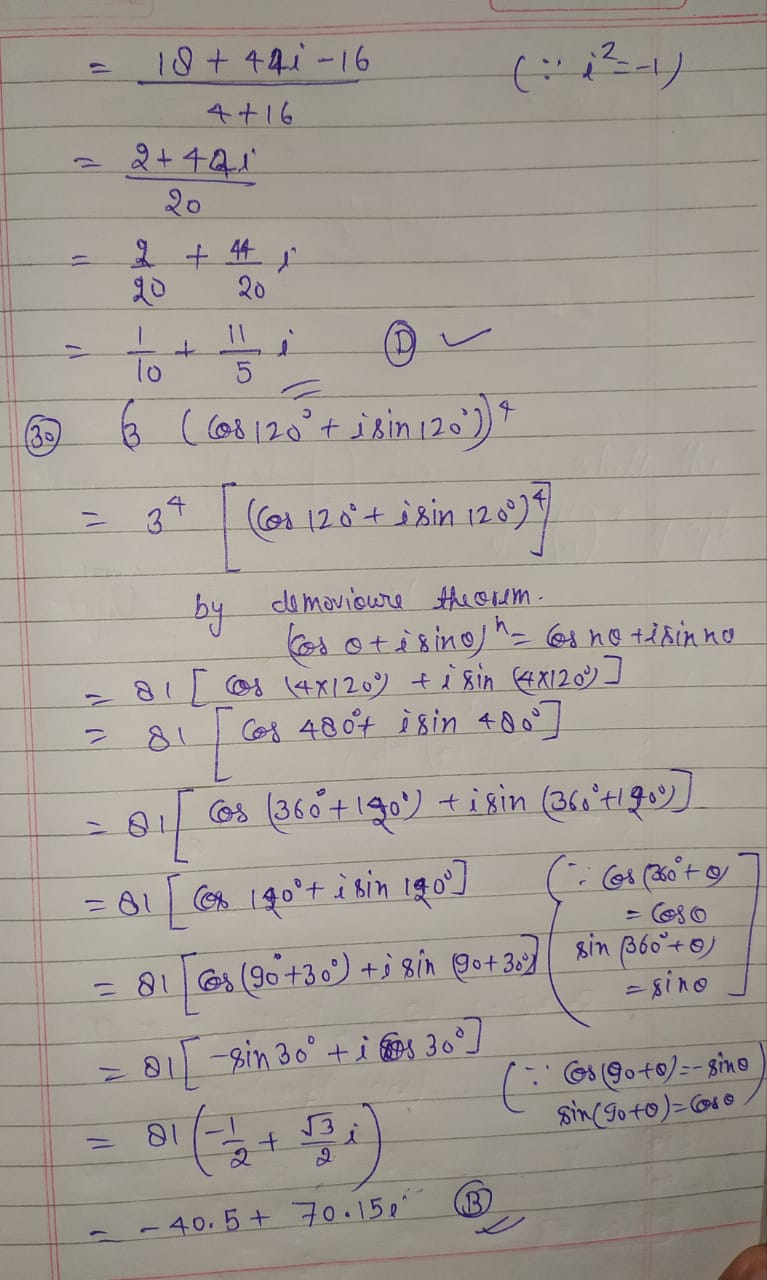Know the answer?
Your Answer:

#### Post as a guest

Your Name:

What's your source?

#### Earn Coin

Coins can be redeemed for fabulous gifts.

Not the answer you're looking for? Ask your own homework help question. Our experts will answer your question WITHIN MINUTES for Free.
Similar Homework Help Questions
• ### Do the indicated operation and write the answer in the complex form a + bi. a)...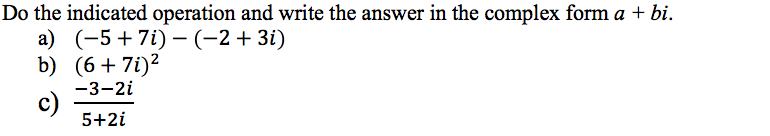Do the indicated operation and write the answer in the complex form a + bi. a) (-5 + 7i)-(-2+ 3i) b) (6 + 7i)2 -3-2i c) 5+2 i

• ### A complex number is a number in the form of a + bi, where a and...

A complex number is a number in the form of a + bi, where a and b are real numbers and i is the square root of negative 1. Design and create a class called Complex for representing complex numbers. This class should have two attributes, a and b, which represent the parts of the complex number. This class should overload the +,-,*,/,++ and -- operators (both prefix and suffix) to perform basic complex number calculations according to the following...

• ### perform the indicated operations. (2-5i)(3-i)-(4-i)(4+i) (simplify your answer. Type your answer in the form a+bi)

perform the indicated operations. (2-5i)(3-i)-(4-i)(4+i) (simplify your answer. Type your answer in the form a+bi)

• ### Perform the indicated operations and write the result in standard form, a +bi. 34 - 25...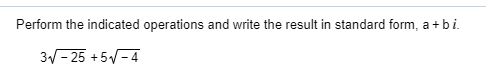Perform the indicated operations and write the result in standard form, a +bi. 34 - 25 +57-4

• ### Perform the indicated operations and write the answer in the form a + bi, where a and b are real numbers

Perform the indicated operations and write the answer in the form a + bi, where a and b are real numbers. Problem #1 (3+2i)^2Problem #2 i^3 - i^2

• ### Simplify and write your answer in the form of a + bi, where a and b...

Simplify and write your answer in the form of a + bi, where a and b are real numbers:   please explain.

• ### Perform the indicated operations. Write the answer in standard form, a+bi. (V2 + 10 i)(12 -...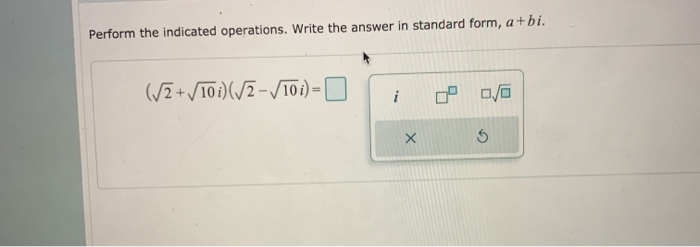Perform the indicated operations. Write the answer in standard form, a+bi. (V2 + 10 i)(12 - 101) 0 0/0 х 5

• ### Use DeMoivre's Theorem to find the indicated power of the complex number. Write the answer in...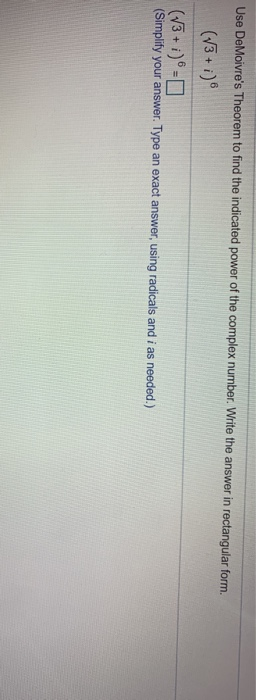Use DeMoivre's Theorem to find the indicated power of the complex number. Write the answer in rectangular form. (V3+ i) (73 +i) (Simplify your answer. Type an exact answer, using radicals and i as needed.) This Question: 1 pt Use the figure below. Determine whether the given statement is true or false. B C A F G D E A+H=F True False Click to select your answer. State whether the vectors are parallel, orthogonal, or neither. v = 4i -...

• ### Write the complex number in the form a + bi. 5) Vocos 315º + i sin...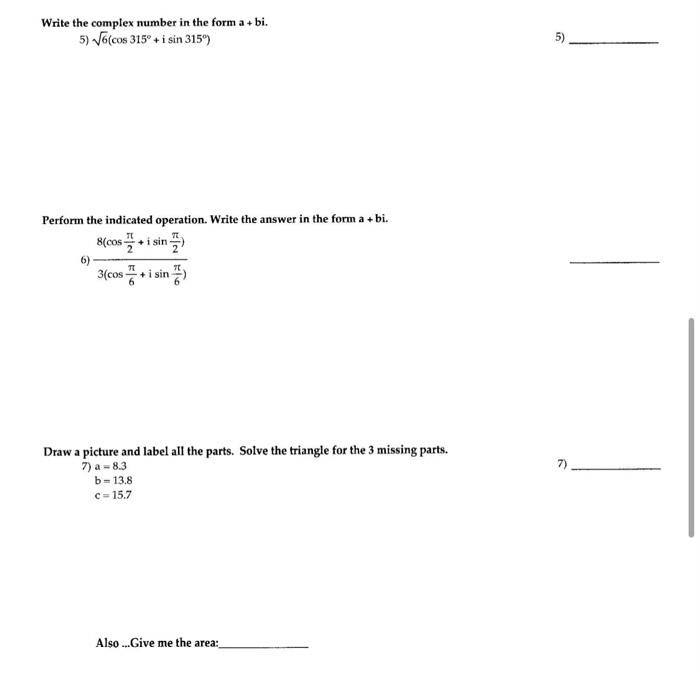Write the complex number in the form a + bi. 5) Vocos 315º + i sin 3159) 5) Perform the indicated operation. Write the answer in the form a +bi. 8(cos i sin 6) 3(cos + i sin 7) Draw a picture and label all the parts. Solve the triangle for the 3 missing parts. 7) a-8.3 b - 13.8 c=15.7 Also ...Give me the area:

• ### JAVA PROGRAMMING A complex number is a number in the form a + bi, where a...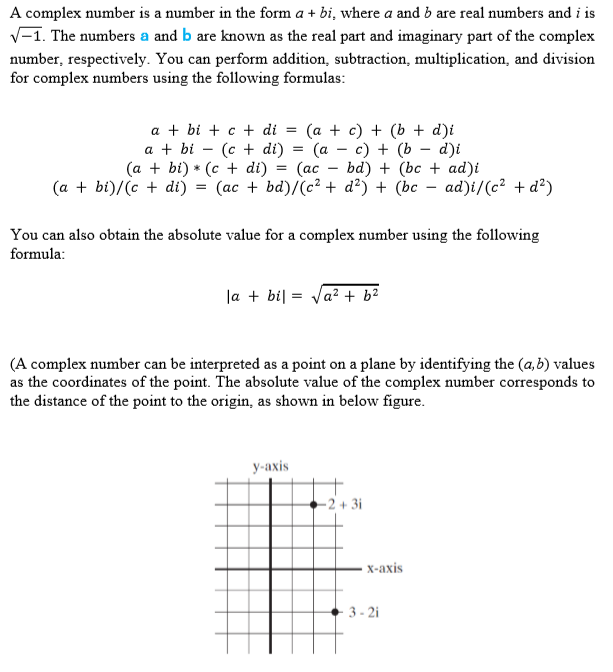JAVA PROGRAMMING A complex number is a number in the form a + bi, where a and b are real numbers and i is V-1. The numbers a and b are known as the real part and imaginary part of the complex number, respectively. You can perform addition, subtraction, multiplication, and division for complex numbers using the following formulas: a + bi + c + di = (a + c) + (b + di a + bi - (c +...

Free Homework App

Scan Your Homework
to Get Instant Free Answers
Need Online Homework Help?

Get Answers For Free
Most questions answered within 3 hours.